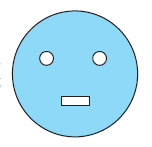# Ex.11.3 Q10 Perimeter and Area - NCERT Maths Class 7

Go back to  'Ex.11.3'

## Question

From a circular card sheet of radius $$14 \rm\,cm$$, two circles of radius $$3.5\rm\, cm$$ and a rectangle of length $$3\rm\, cm$$ and breadth $$1 \rm\,cm$$ are removed. (as shown in the adjoining figure). Find the area of the remaining sheet. (Take $$π =22/7$$ )Video Solution
Perimeter And Area
Ex 11.3 | Question 10

## Text Solution

What is known?

From a circular card sheet of radius $$14 \rm\,cm$$, two circles of radius $$3.5\rm\, cm$$ and a rectangle of length $$3\rm\, cm$$ and breadth $$1 \rm\,cm$$ are removed.

What is unknown?

The area of the remaining sheet.

Reasoning:

From a circular card sheet of radius $$14 \rm\,cm$$,two circles of radius $$3.5\rm\, cm$$ and a rectangle of length $$3\rm\, cm$$ and breadth $$1 \rm\,cm$$ are removed. To find the area of the remaining card sheet, first find the area of the circular sheet then the area of the two circles of radius $$3.5\rm\, cm$$ and a rectangle of length $$3\rm\, cm$$ and breadth $$1 \rm\,cm$$. Then subtract the area of the three figures from the area of the circular sheet to get the area of the remaining sheet.

Steps:

Radius of circular sheet $$(R)$$ $$=$$ $$14 \rm\,cm$$ and radius of smaller circle (r) $$=$$ $$3.5\rm\, cm$$

Length of rectangle $$(l)$$ $$=$$ $$3\rm\, cm$$ and breadth of rectangle (b) $$=$$ $$1 \rm\,cm$$

Area of the remaining sheet = Area of circular sheet - Area of two smaller circle + Area of rectangle

\begin{align}&=\! \pi {R^2} \!-\! \left[ {2\pi {r^2} \!+\! \left( {{\rm{Length}} \!\times\! {\rm{Breadth}}} \right)} \right]\\ &=\! \frac{{22}}{7} \!\times\! 14 \!\times\! 14\! - \!\left[ {2 \!\times\! \frac{{22}}{7} \!\times\! 3.5 \!\times\! 3.5 \!+\! \left( {3 \!\times\! 1} \right)} \right]\\ &=\! 22 \!\times\! 2 \!\times\! 14\! -\! \left[ {\frac{{44}}{7} \!\times\! 3.5 \!\times\! 3.5 \!+\! 3} \right]\\ &\!= 616 \!-\! \left( {22 \!\times\! 3.5 \!+\! 3} \right)\\ &=\! 616 \!-\! \left( {77 \!+\! 3} \right)\\ &=\! 616 \!- \!80\\ &= \!536\;\rm c{m^2}\end{align}

Therefore, the area of remaining sheet is $$536 \rm\,cm^2.$$

Learn from the best math teachers and top your exams

• Live one on one classroom and doubt clearing
• Practice worksheets in and after class for conceptual clarity
• Personalized curriculum to keep up with school